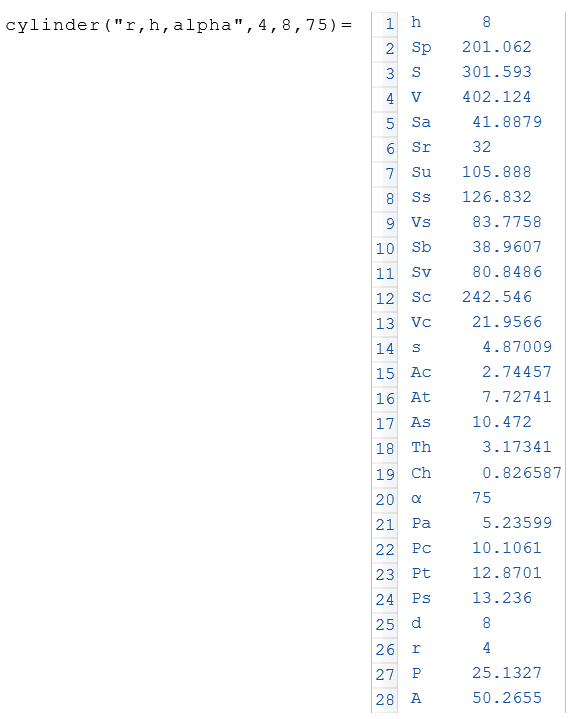# Class Cylinder

Calculation of the properties of cylinders

## Description

The class Cylinder calculates properties of a cylinder, sector or section.

## Properties

The following properties can be queried as a result or passed as an argument.

#### Description

Basics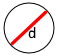d Diameter of the base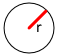r Radius of the base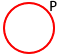P Circumference of the base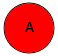A Area of the base
Cylinder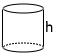h Height of the cylinder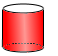Sp Surface without top and base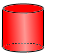S Total surfaceV Volume of the cylinder
Sector of the base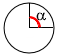$$α$$ Sector angle alpha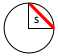s Length of chord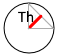Th Height of the triangleCh Height of the segment
Area of the sectorAs Area of the base sector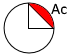Ac Area of the base segment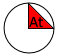At Area of the base triangle
Segment and Sector PerimeterPc Perimeter of th segment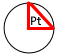Pt Perimeter of the triangle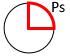Ps Perimeter of the sector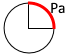Pa Length of the arc
Cylinder Sector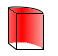Sa Surface of the cylinder arcSr Surface of one even side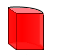Su Sector surface without top and base (2 Sr + Sa)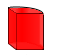Ss Sector surface (2 As + Su) include top and baseVs Sector volume
Cylinder Segment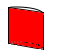Sb Even surface of the cylinder chord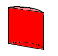Sv Surface of the cylinder segments chord and arc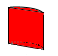Sc Surface of the cylinder segment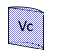Vc Volume of the cylinder segment

## Syntax

Object = Cylinder ("arg names", argumente)

Value = Cylinder ("get -> arg names", argumente)

## Examples

The following example specifies a radius $$r$$ of 4 and a height $$h$$ of 8 as arguments.The following example displays the volume $$V$$ of the object $$a$$.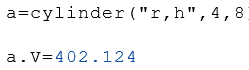In the following example, the property $$V$$ is calculated from the radius $$r$$ and the height $$h$$.If, apart from $$r$$ and $$h$$, the angle alpha is specified, all properties can be calculated.